## Example Questions

1 2 3 4 5 6 7 8 10 Next →

### Example Question #91 : Statistics

Find the mode of the following set of numbers: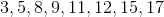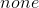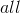Explanation:

To find mode, simply find the number that occurs most frequently.

Since there isn't a number that occurs more than once, there is no mode.

### Example Question #101 : Data Analysis

Find the mode of the following set of numbers: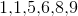Explanation:

To find mode, simply find the most frequent number. Thus, you answer is.

### Example Question #1 : Range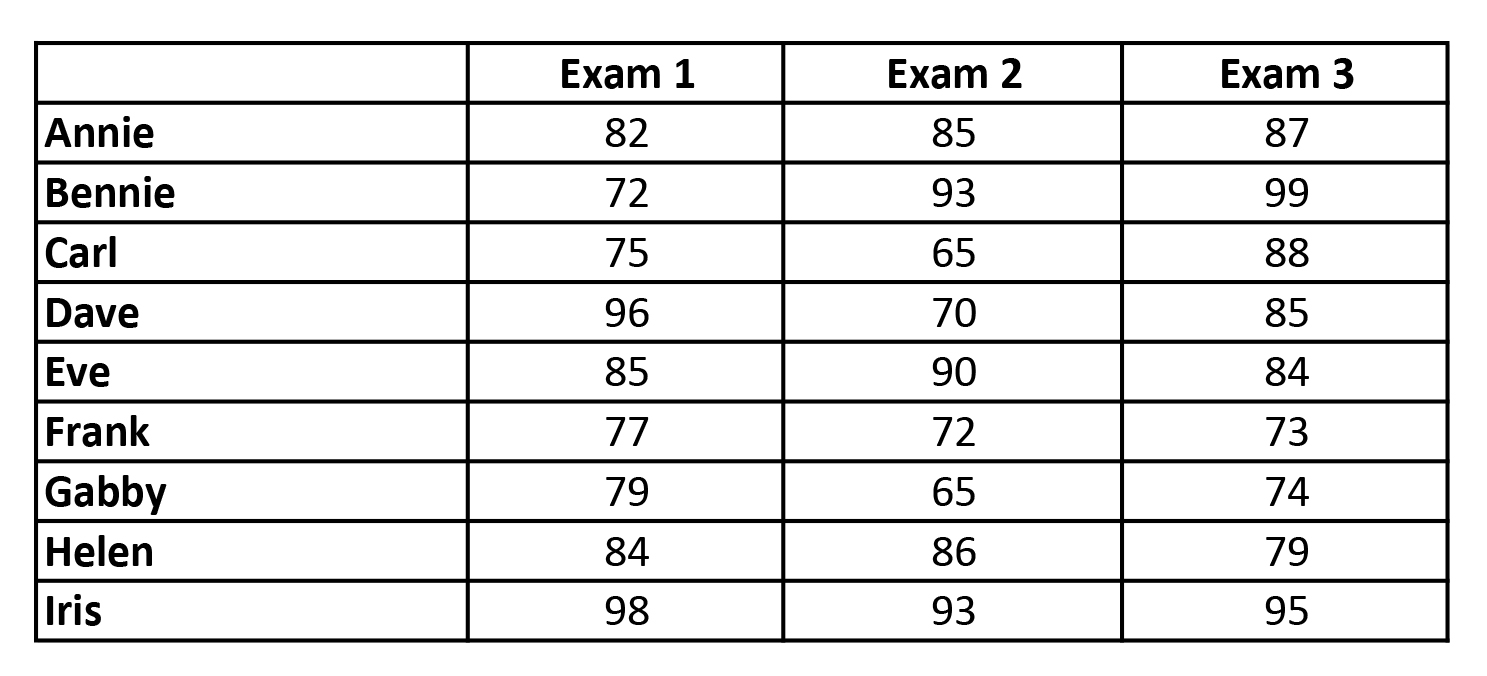What is the range of the grades Helen received on all three exams?

7

12

82

84

9

7

Explanation:

The range of a set of data is the difference between the highest and lowest values in the set.

Highest = 86

Lowest = 79

Range = 86 - 79 = 7

### Example Question #2 : Range

If train A heads from Albuquerque, New Mexico towards Phoenix, Arizona, at 50 miles an hour and train B heads from Phoenix to Albuquerque at 70 miles an hour leaving at the same time at 4:00PM, at what time will the trains intersect taking the same path, assuming the distance between the two cities is 450 miles.

Between 8:00 and 9:00 PM

Between 7:00 and 8:00 PM

Between 6:00 and 7:00 PM

Between 9:00 and 10:00 PM

Between 7:00 and 8:00 PM

Explanation:

A quick look at the answer choices tells you that the question is not looking for the exact time of intersection, but rather between what two hours the trains will intersect. The best approach to a problem like this is to quickly make a table listing distance traveled of each train and the corresponding time. An example is illustrated below.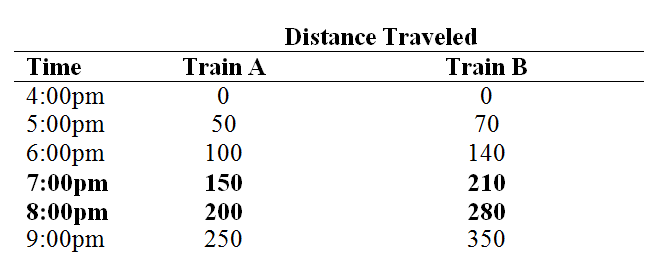We see at 7:00 PM the trains have not yet intersected (and are actually 90 miles apart,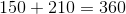|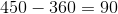miles.

At 8:00 PM the trains have traveled a total of 480 miles together, and thus must have already intersected. Therefore, between 7:00 and 8:00 PM is the correct answer.

Questions such as these should take no longer than twenty seconds, as they only require simple arithmetic. They tend to be more intimidiating than difficult, as well as many other questions on the ACT.

### Example Question #1 : Range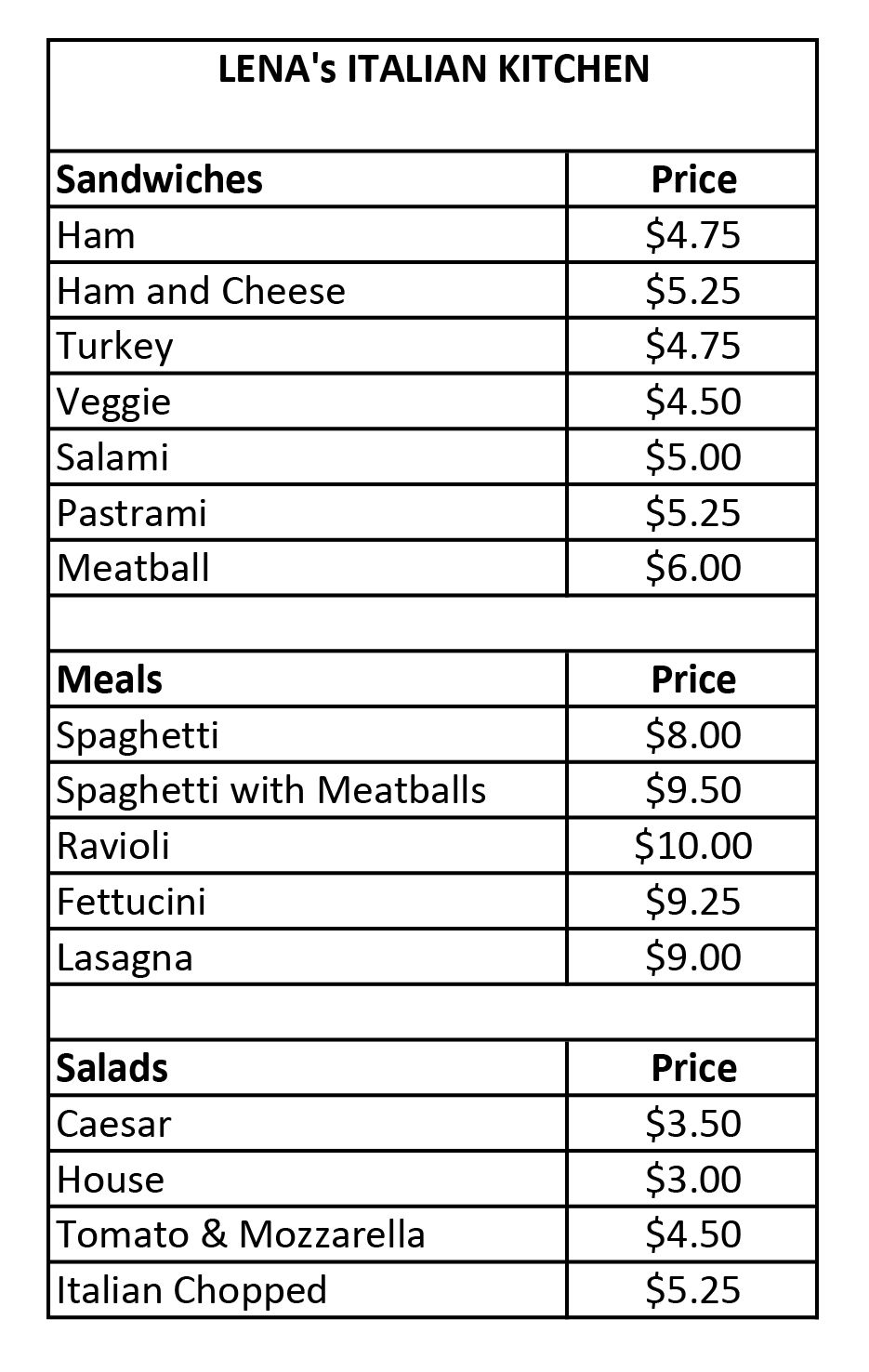Considering the entire menu shown for Lena’s Italian Kitchen, what is the range of the prices of the many food options?

$3.50$4.50

$6.09$1.50

$7.00 Correct answer:$7.00

Explanation:

The range is difference between the largest value and the smallest value.

The largest value is the ravioli for $10.00 The smallest value is the house salad for$3.00

The range = $10.00 -$3.00 = \$7.00

### Example Question #2 : Range

Find the range of the following set of numbers: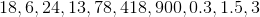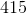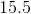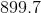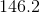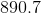Explanation:

Range is the difference between the largest number in the set and the smallest number in the set. Our largest number in the set is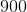and our smallest number is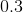.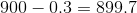### Example Question #91 : Statistics

The following is a list of the amount of students in each of five 3rd grad classrooms:
23, 27, 19, 31, 33. What is the range of the number of students in each classroom?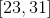Explanation:

The range is the highest minus the lowest value in a given data set contains. Thus the low end of the range is the low number, or 19 students, and the high end of the range is the high number, or 33.

Subtracting the lowest value from the highest we get,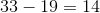.

Therefore the range of the data set is.

### Example Question #1 : How To Find Range

Find the range of the following set of numbers.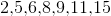Explanation:

To find range, simply subtract the smallest number from the largest number. Thus,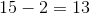### Example Question #7 : Range

Find the range of the following set of numbers:Explanation:

To find the range, simply take the difference of the smallest and largest numbers.

The largest number is,and the smallest number is.

Thus,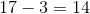.

### Example Question #1 : Range

Find the range of the following set of numbers: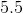Explanation:

To find range, simply subract the smallest number from the largest. Thus,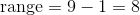1 2 3 4 5 6 7 8 10 Next →

### All ACT Math Resources